##### Algebra II Workbook For DummiesIn algebra, you often come across equations that have no real solutions — or equations that have the potential for many more real solutions than they actually have. For instance, the equation x2 + 1 = 0 has no real solutions. If you write it as x2 = –1 and try to take the square root of each side, you run into trouble.

Not until you have the imaginary numbers can you write that the solution of this equation is x = +/–i. The equation has two complex solutions.

An example of an equation without enough real solutions is x4 – 81 = 0. This equation factors into (x2 – 9)(x2 + 9) = 0. The two real solutions of this equation are 3 and –3. The two complex solutions are 3i and –3i.

To solve for the complex solutions of an equation, you use factoring, the square root property for solving quadratics, and the quadratic formula.

## Sample questions

1. Find all the roots, real and complex, of the equation x3 – 2x2 + 25x – 50 = 0.

x= 2, 5i, –5i. First, factor the equation to get x2(x – 2) + 25(x – 2) = (x – 2)(x2 + 25) = 0. Using the multiplication property of zero, you determine that x – 2 = 0 and x = 2. You also get x2 + 25 = 0 and x2 = –25. Take the square root of each side, and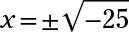Simplify the radical, using the equivalence for i, and the complex solutions are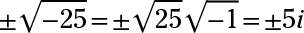The real root is 2, and the imaginary roots are 5i and –5i.

2. Find all the roots, real and imaginary, of the equation 5x2 – 8x + 5 = 0.

x = 0.4 + 0.6i, 0.4 – 0.6i. The quadratic doesn’t factor, so you use the quadratic formula: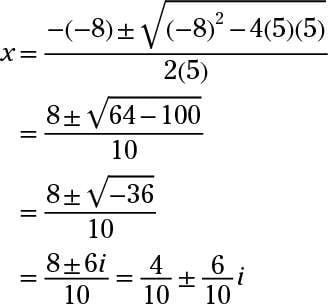The only two solutions are complex: 0.4 + 0.6i and 0.4 – 0.6i.

## Practice questions

1. Find all the roots, real and imaginary, of x2 + 9 = 0.

2. Find all the roots, real and imaginary, of x2 + 4x + 7 = 0.

3. Find all the roots, real and imaginary, of 5x2 + 6x + 3 = 0.

4. Find all the roots, real and imaginary, of x4 + 12x2 – 64 = 0.

Following are answers to the practice questions:
1. The answer is x = 3i, –3i.

Add –9 to each side to get x2 = –9. Take the square root of each side. Then simplify the expression using i for the negative under the radical: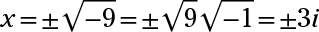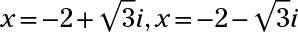Use the quadratic formula to solve for x. Simplify the expression using i for the negative under the radical: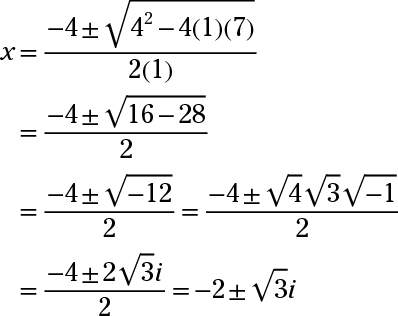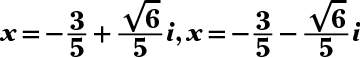Use the quadratic formula to solve for x. Simplify the expression using i for the negative under the radical: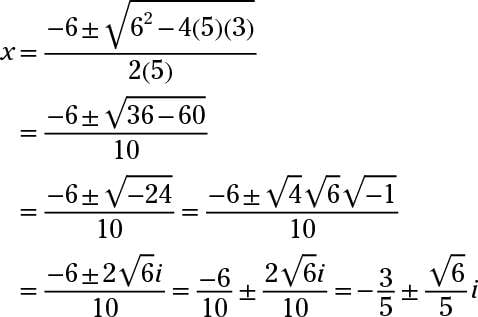4. The answer is x= 2, –2, 4i, –4i.

Factor the left side: (x2 + 16)(x2 – 4) = (x2 + 16)(x – 2)(x + 2) = 0. Obtain the two real roots by setting x – 2 and x + 2 equal to 0. When x2 + 16 = 0, you find that x2 = –16. Taking the square root of each side and using i for the –1 under the radical gives you the two imaginary roots.